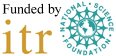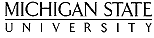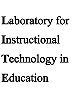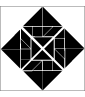When you take a LON-CAPA created randomized Scantron exam, you are given partial credit for option response problems. LON-CAPA will show a yellow check-minus graphic next to the problems that are partially correct on the Navigation screen. View the problem to determine your partial credit.

## Example scored option response problem that is 50% correct:

Identify the statements as being either True or False.
True: A dimensionally incorrect equation must be wrong.
 A: True B: False

False: A dimensionally correct equation must be correct.
 A: True B: False

 You are partially correct. Computer's answer now shown above.Your receipt is 157-2320## How to read the feedback (notice highlighed True and False):

Identify the statements as being either True or False.
True: A dimensionally incorrect equation must be wrong.
 A: True B: False

False: A dimensionally correct equation must be correct.
 A: True B: False

 You are partially correct. Computer's answer now shown above.Your receipt is 157-2320